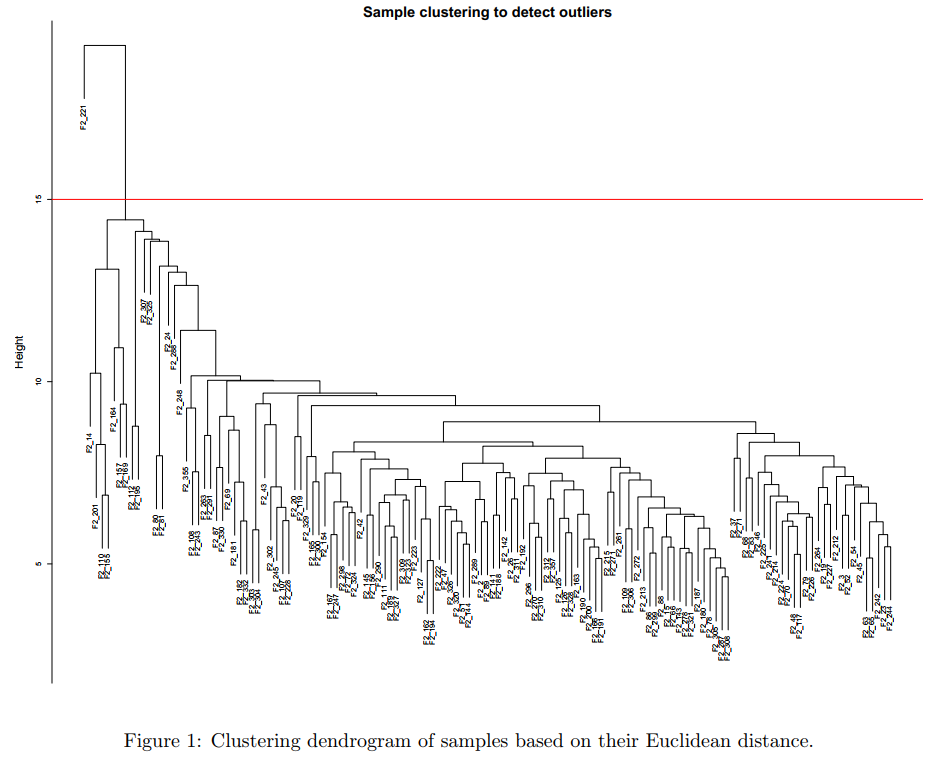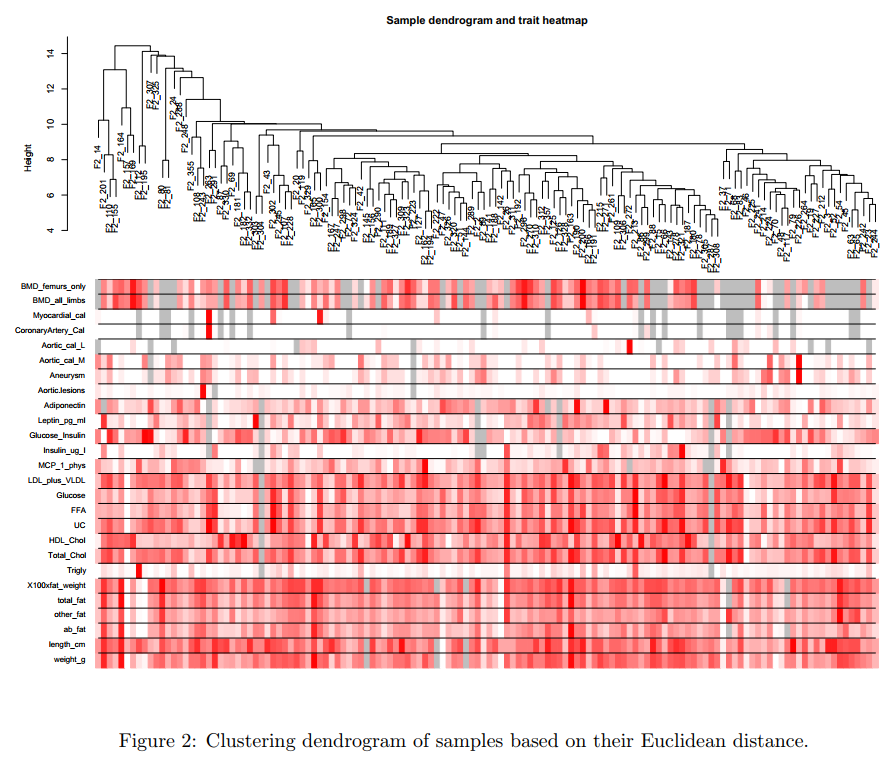# 1. 数据导入与清理

## 1.1. 样本-表达数据获取

### 1.1.1. 导入数据

``````# 设定工作目录
setwd("/home/cxt/data/R/")
# 导入包
library(WGCNA)

# 设置将strings不要转成factors
options(stringsAsFactors=F)

# 读入当前目录中的表达数据
# 查看数据的行列个数以及列名
dim(femData)
names(femData)
``````

### 1.1.2. 提取表达数据

``````# 提取出表达数据
datExpr0 <- as.data.frame(t(femData[, -c(1:8)]))
names(datExpr0) <- femData\$substanceBXH
rownames(datExpr0) <- names(femData)[-c(1:8)]
``````

### 1.1.3. 离群样本清理

``````## 第一步：样本or基因缺失值情况评估
# 在列表中的基因或者样本中缺失值是否很多
gsg <- goodSamplesGenes(datExpr0, verbose=3)
# 是true的话说明所有基因和样本都通过了筛选，否则需要删除不符合标准的样本和基因
gsg\$allOK
if (!gsg\$allOK){
if (sum(!gsg\$goodGenes)>0)
printFlush(paste("Removing genes:", paste(names(datExpr0)[!gsg\$goodGenes], collapse=", ")))
if (sum(!gsg\$goodSamples)>0)
printFlush(paste("Removing samples:", paste(rownames(datExpr0)[!gsg\$goodSamples], collapse=", ")))
datExpr0 <- datExpr0[gsg\$goodSamples, gsg\$goodGenes]
}

## 第二步：样本聚类检查离群值评估
# 画样本树状图，看是否有特别离群的样本
sampleTree <- hclust(dist(datExpr0), method="average")
sizeGrWindow(12, 9)
par(cex=0.6)
par(mar=c(0,4,2,0))
plot(sampleTree, main="Sample clustering to detect outliers", sub="",
xlab="", cex.lab=1.5,
cex.axis=1.5, cex.main=2)
````````````# 在确认离群样本后，我们可以手动删除数据，或者自动删除数据（下面的代码）
# 首先利用纵坐标的值，画出一个红线，以区分出离群样本
abline(h=15, col="red")
# 获取位于红线下方的样本信息
clust <- cutreeStatic(sampleTree, cutHeight=15, minSize=10)
table(clust)
# clust等于1的样本就是我们要保留的
keepSamples <- (clust==1)
datExpr <- datExpr0[keepSamples, ]
nGenes <- ncol(datExpr)
nSamples <- nrow(datExpr)
``````

## 1.2. 样本-临床信息获取

``````# 导入数据
dim(traitData)
names(traitData)

# 只保留我们需要的表型信息
allTraits <- traitData[, -c(31, 16)]
allTraits <- allTraits[, c(2, 11:36)]
dim(allTraits)
names(allTraits)

# 使两个数据框（表达与临床）的行名一致
femaleSamples <- rownames(datExpr)
traitRows <- match(femaleSamples, allTraits\$Mice)
datTraits <- allTraits[traitRows, -1]
rownames(datTraits) <- allTraits[traitRows, 1]

# 清理，释放内存
collectGarbage()
``````

## 1.3. 样本-表达-临床关联分析

``````# 作性状与样本的关系热图
sampleTree2 <- hclust(dist(datExpr), method="average")
traitColors <- numbers2colors(datTraits, signed=F)
plotDendroAndColors(sampleTree2, traitColors,
groupLabels=names(datTraits),
main="Sample dendrogram and trait heatmap")

# 保存预处理完成的数据
save(datExpr, datTraits, file="dataInput.RData")
``````## 1.4. 保存预处理完成的数据

``````save(datExpr, datTraits, file="dataInput.RData")
``````

### 评论

###### Your browser is out of date!

Update your browser to view this website correctly. Update my browser now

×